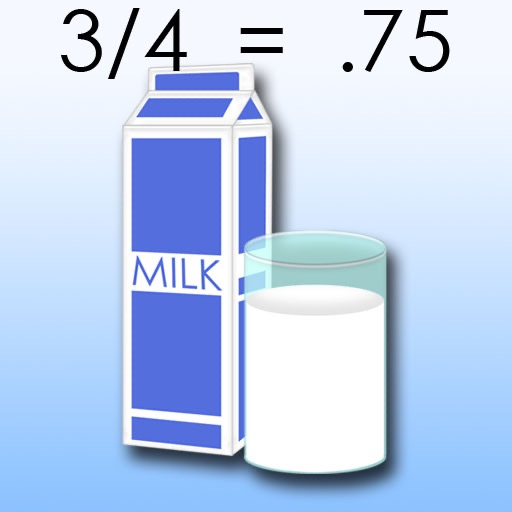# Portion Platter (Fraction)

Draw a line across a glass of milk to match the given fraction for the first problem type. For the second problem type, a fractional portion is displayed as a cookie, peppermint candy, pie or pizza and you enter the matching fraction or percentage. Good Luck!
Category Price Seller Device
Education \$0.99 new river community college games iPhone, iPad, iPod

Portion Platter (Fractions) helps students better visualize fractions and percentages and equivalent relationships between them. The touch mechanic engages children in a hands-on learning process, implementing kinesthetic learning. Two types of problems are presented at 4 different difficulty levels appropriate for grades 2-6.
The basic idea of Portion Platter is enable children to better visualize fractions.

Also play other NRCC games:
Same Sound Spellbound (homophones)
Opposite Ocean (antonyms)
Grammar Dragon (parts of speech)
Factor Race (binomial and trinomial equations)
Spelling Cat (spelling)
Same Meaning Magic (synonyms)

Contact: [email protected]

FOR TEACHERS AND PARENTS:

The recommended graded level are as follows:

The game incorporates learning fractions attuned to the particular grade level, based upon excerpts from grade-appropriate math materials.

All components of the game are developed in-house. The Virginia Standards of Learning targeted are for:

MATH

2.4
The student will identify the part of a set and/or region that represents fractions for one- half, one-third, one-fourth, one-eighth, and one-tenth and write the corresponding fraction.

3.5
a)divide regions and sets to represent a fraction; and
b)name and write the fractions represented by a given model (area/region, length/measurement, and set). Fractions (including mixed numbers) will include halves, thirds, fourths, eighths, and tenths.

3.6
The student will compare the numerical value of two fractions having like and unlike denominators, using concrete or pictorial models involving areas/regions, lengths/measurements, and sets.

4.2
a) identify, model, and compare rational numbers (fractions and mixed numbers), using concrete objects and pictures;
b) represent equivalent fractions;
c) and relate fractions to decimals, using concrete objects.

4.3
The student will compare the numerical value of fractions (with like and unlike denominators) having denominators of 12 or less, using concrete materials.

5.2
a)recognize and name commonly used fractions (halves, fourths, fifths, eighths, and tenths) in their equivalent decimal form and vice versa;

6.1
The student will identify representations of a given percent and describe orally and in writing the equivalence relationships among fractions, decimals, and percents.

CREDITS
This game is made possible with an Enhancing Education Through Technology competitive grant from the Virginia Department of Education.

### Reviews

Reviews not available.